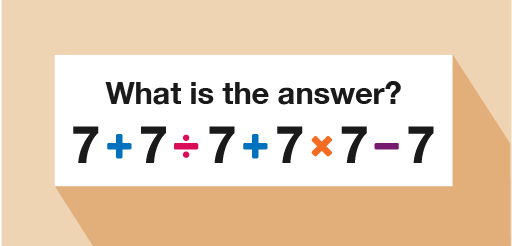Science, Maths & Technology

### Become an OU studentEveryday maths 2

Start this free course now. Just create an account and sign in. Enrol and complete the course for a free statement of participation or digital badge if available.

# 8 FormulasFigure _unit2.8.1 Figure 20 Formulas

Before diving in to this topic, you first need to learn about the order in which you need to carry out operations. Have you ever seen a question like the one below posted on social media?Figure _unit2.8.2 Figure 21 A calculation using the four operations

There are usually a wide variety of answers given by various people. But how is it possible that such a simple calculation could cause so much confusion? It’s all to do with the order in which you carry out the calculations.

• If you go from left to right:

• 7 + 7 = 14

• 14 ÷ 7 = 2

• 2 + 7 = 9

• 9 × 7 = 63

• 63 − 7 = 56

The correct answer however, is actually 50. How do you arrive at this answer? You have to use the correct order of operations, sometimes called BIDMAS.